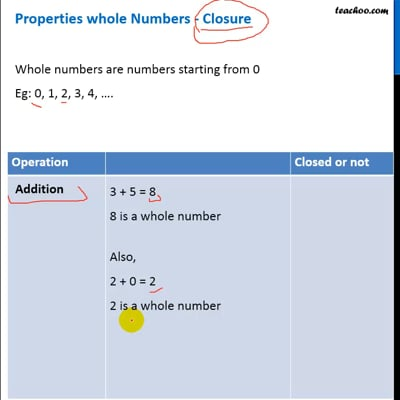Properties of whole numbers

Chapter 2 Class 6 Whole Numbers
Concept wise

### Properties whole Numbers - Closure

Whole numbers are numbers starting from 0

Eg: 0, 1, 2, 3, 4, ….

 Operation Closed or not Addition 3 + 5 = 8 8 is a whole number Also, 2 + 0 = 2 2 is a whole number So, whole numbers are closed under addition So, if we add any two numbers, we get a whole number So, it is closed Subtraction 3 – 5 = –2 But, –2 is not a whole number So, whole number are not closed under subtraction So, if we subtract any two numbers, we do not get a whole number So, it is not closed Multiplication 3 × 5 = 15 15 is a whole number Also, 2 × 0 = 0 0 is a whole number So, whole numbers are closed under multiplication So, if we multiply any two numbers, we get a whole number So, it is closed Division 3 ÷ 5 = 3/5 But, 3/5 is not a whole number So, whole number are not closed under division So, if we divide any two numbers, we do not get a whole number So, it is not closed

Hence,

Whole numbers are closed under Addition and Multiplication

but not under Subtraction and DivisionThis video is only available for Teachoo black users

### Transcript

Properties whole Numbers - Closure Whole numbers are numbers starting from 0 Eg: 0, 1, 2, 3, 4, …. 3 + 5 = 8 8 is a whole number Also, 2 + 0 = 2 2 is a whole number So, whole numbers are closed under addition So, if we add any two numbers, we get a whole number So, it is closed 3 – 5 = –2 But, –2 is not a whole number So, whole number are not closed under subtraction So, if we subtract any two numbers, we do not get a whole number So, it is not closed 3 × 5 = 15 15 is a whole number Also, 2 × 0 = 0 0 is a whole number So, whole numbers are closed under multiplication So, if we multiply any two numbers, we get a whole number So, it is closed 3 ÷ 5 = 3/5 But, 3/5 is not a whole number So, whole number are not closed under division So, if we divide any two numbers, we do not get a whole number So, it is not closed Hence, Whole numbers are closed under Addition and Multiplication but not under Subtraction and Division